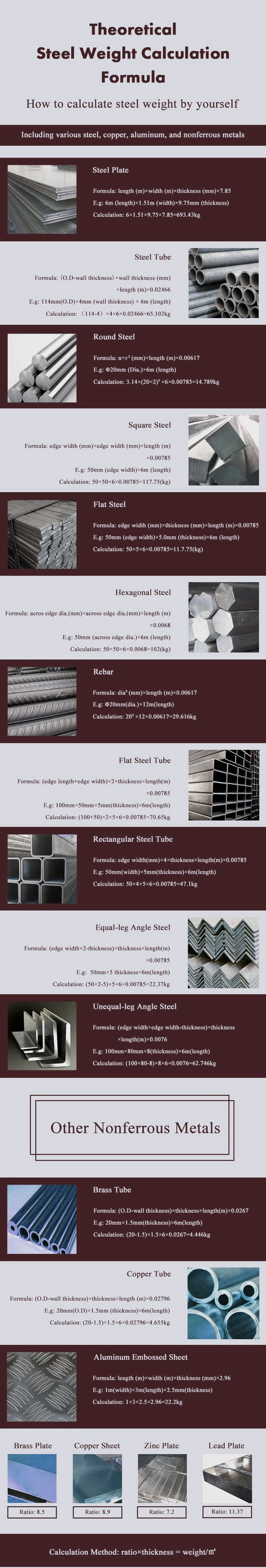# Steel Weight Caculator

#### MPJain » Steel Weight Caculator# Related Various Theoretical Metal Weight Calculation Formula

Octagonal Steel (kg)=0.0065×across width×across width×length
Round Aluminum Rod (kg)=0.0022×dia.×dia.×length
Square Red Copper Rod (kg)=0.0089×width×width×length
Copper Plate (kg)=0.0089×thickness×width×length
Brass Plate (kg)=0.0085×thickness×width×length
Aluminum Plate (kg)=0.00171×thickness×width×length
Flat Steel (kg)=0.00785×thickness×width×length
Square Brass Copper Rod (kg)=0.0085×width×width×length
Square Aluminum Rod (kg)=0.0028×width×width×length
Hexagonal Aluminum Rod (kg)=0.00242×across width×across width×length
Round Red Copper Tube (kg)=0.028×thickness×(O.D-thickness)×length
Round Brass Tube (kg)=0.0267×thickness×(O.D-thickness)×length
Round Copper Rod (kg)=0.00698×dia.×dia.×length
Round Brass Rod (kg)=0.00668×dia.×dia.×length
Hexagonal Copper Rod (kg)=0.0077×across width×across width×length
Hexagonal Brass Rod (kg)=0.00736×width×across width×length
Round Aluminum Copper Tube (kg)=0.00879×thickness×(O.D-thickness)×length

Note: in above formula, the unit of length is “m”，unit of area is “㎡”，the rest unit is “mm”